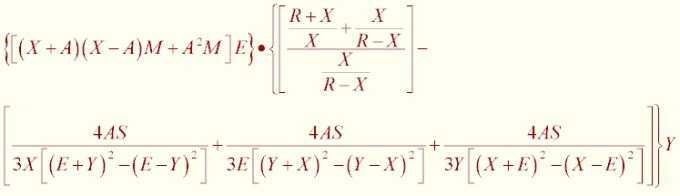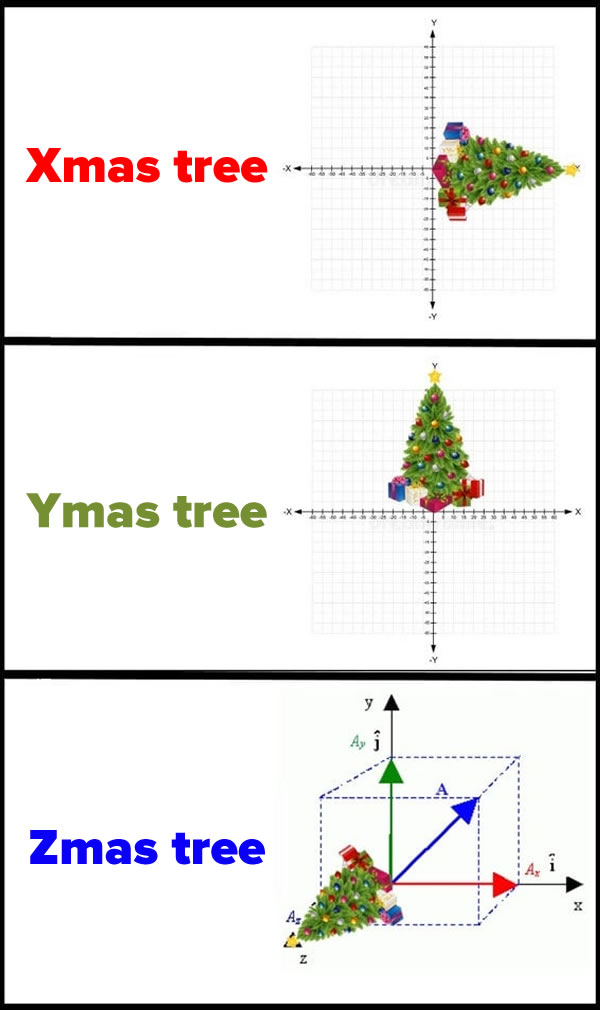## Poll

 I love math!17 votes (50%) Math is great.13 votes (38.23%) My religion is mathology.5 votes (14.7%) Women didn't speak to me until I was 30.2 votes (5.88%) Total eclipse reminder -- 04/08/202411 votes (32.35%) I steal cutlery from restaurants.3 votes (8.82%) I should just say what's on my mind.6 votes (17.64%) Who makes up these awful names for pandas?5 votes (14.7%) I like to touch my face.10 votes (29.41%) Pork chops and apple sauce.8 votes (23.52%)

34 members have voted

chevyJoined: Apr 15, 2011
• Posts: 89
December 21st, 2020 at 10:28:16 PM permalink
Quote: ssho88

a) a = 2*(pi)^0.5/3^0.25 * r = 2.693547r
b) Total Area outside circle = 0.573249r^2

BUT....

You are CORRECT!!!

(Sorry, just flashing back to high school there.)

What did the triangle say to the circle?

You're pointless!

GialmereJoined: Nov 26, 2018
• Posts: 2104
December 22nd, 2020 at 4:47:42 PM permalink
A belated tannenbaum Tuesday...Place each of the numbers from 1 to 10 on the tree ornaments so that every line adds up to the same number.

There are five lines, three lines containing four ornaments, one line with three ornaments, and one line with just two ornaments.
Have you tried 22 tonight? I said 22.
ssho88Joined: Oct 16, 2011
• Posts: 552
Thanks for this post from:December 22nd, 2020 at 7:42:39 PM permalink
Quote: Gialmere

A belated tannenbaum Tuesday...Place each of the numbers from 1 to 10 on the tree ornaments so that every line adds up to the same number.

There are five lines, three lines containing four ornaments, one line with three ornaments, and one line with just two ornaments.

There are 3 rows and total of those 10 numbers is 55, so each row add up should be either 18, 17, 16 or 15. Only 18 is possible.

1
10 8
5 7 6
2 9 4 3

GialmereJoined: Nov 26, 2018
• Posts: 2104
December 22nd, 2020 at 8:38:58 PM permalink
Quote: ssho88

There are 3 rows and total of those 10 numbers is 55, so each row add up should be either 18, 17, 16 or 15. Only 18 is possible.

1
10 8
5 7 6
2 9 4 3

Correct!

There's also a few mirror image variants that work.

-----------------------------Have you tried 22 tonight? I said 22.
GialmereJoined: Nov 26, 2018
• Posts: 2104
December 23rd, 2020 at 8:03:18 AM permalinkSimplify the following equation...Have you tried 22 tonight? I said 22.
ThatDonGuyJoined: Jun 22, 2011
• Posts: 4957
Thanks for this post from:December 23rd, 2020 at 8:59:41 AM permalink
Quote: Gialmere

Simplify the following equation...The first part is:
((X + A)(X - A) M + A^2 M) E
= M (X^2 - A^2 + A^2) E
= M E X^2

The left side of the second part is:
((R + X) / X + X / (R - X)) / (X / (R - X))
= ((R^2 - X^2 + X^2) / (X (R - X))) / (X / (R - X))
= R^2 / (X (R - X)) * (R - X) / X
= R^2 / X^2

The right side of the second part is:
4AS / 3 * (1 / (X ((E + Y)^2 - (E - Y)^2)) + 1 / (E ((Y + X)^2 - (Y - X)^2)) + 1 / (Y ((X + E)^2 - (X - E)^2)))
= 4AS / 3 * (1 / 4XEY + 1 / 4EYX + 1 / 4YXE)
= 4AS / 3 * 3/4 * 1/XEY
= AS / XEY

Subtract the right side of the second part from the left side, then multiply by Y:
Y (R^2 / X^2 - AS / XEY)
= Y (R^2 XEY - AS X^2) / (X^3 EY)
= (R^2 EY - ASX) / (X^2 E)

The product of the two parts is:
MEX^2 (R^2 EY - ASX) / (X^2 E)
= M (R^2 EY - ASX)
= MERRY - XMAS

GialmereJoined: Nov 26, 2018
• Posts: 2104
December 23rd, 2020 at 4:37:56 PM permalink
Quote: ThatDonGuy

The first part is:
((X + A)(X - A) M + A^2 M) E
= M (X^2 - A^2 + A^2) E
= M E X^2

The left side of the second part is:
((R + X) / X + X / (R - X)) / (X / (R - X))
= ((R^2 - X^2 + X^2) / (X (R - X))) / (X / (R - X))
= R^2 / (X (R - X)) * (R - X) / X
= R^2 / X^2

The right side of the second part is:
4AS / 3 * (1 / (X ((E + Y)^2 - (E - Y)^2)) + 1 / (E ((Y + X)^2 - (Y - X)^2)) + 1 / (Y ((X + E)^2 - (X - E)^2)))
= 4AS / 3 * (1 / 4XEY + 1 / 4EYX + 1 / 4YXE)
= 4AS / 3 * 3/4 * 1/XEY
= AS / XEY

Subtract the right side of the second part from the left side, then multiply by Y:
Y (R^2 / X^2 - AS / XEY)
= Y (R^2 XEY - AS X^2) / (X^3 EY)
= (R^2 EY - ASX) / (X^2 E)

The product of the two parts is:
MEX^2 (R^2 EY - ASX) / (X^2 E)
= M (R^2 EY - ASX)
= MERRY - XMAS

Correct!
---------------------------Have you tried 22 tonight? I said 22.
WizardJoined: Oct 14, 2009
• Posts: 23428
December 25th, 2020 at 3:34:23 PM permalink
If G's puzzle from Dec 23 was too hard, perhaps this one will be more to your liking. What does it say?It's not whether you win or lose; it's whether or not you had a good bet.
chevyJoined: Apr 15, 2011
• Posts: 89
December 26th, 2020 at 10:22:40 AM permalink

My belated guess is

me^(rr y) = x-mas

WizardJoined: Oct 14, 2009
• Posts: 23428
December 26th, 2020 at 12:04:40 PM permalink
Quote: chevy

My belated guess is

me^(rr y) = x-mas

Where does the natural log fit into it?
It's not whether you win or lose; it's whether or not you had a good bet.